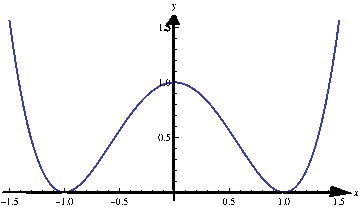# Maximum and Minimum of a Function

## Local maximum and minimum

Consider the function $$f(x)=x^4-2x^2+1$$. Without using too much theory we can express $$f$$ in the following way: $f(x)=\left(x^2-1\right)^2=(x-1)^2\cdot (x+1)^2.$ From this expression we see that the function is always non-negative and its minimum $$0$$ is attained at $$2$$ points: $$-1$$ and $$1$$. On the real line, the function does not have maximum, because as $$x$$ approaches infinity, the value of $$f$$ goes to infinity.

Let us now take a look at the graph of the function $$f$$:The graph shows that the minimum is $$0$$ at two points: $$-1$$ and $$1$$. However, the graph shows that the point $$x=0$$ is special: the function has a peak’’ at that point. It is not real maximum (because we saw that the function goes to $$+\infty$$). This point is called local maximum.’’ We are now going to define this term precisely.

Definition (Local maximum) The function $$f$$ attains a local maximum at point $$a$$ if there is an interval of the form $$(p,q)$$ that contains $$a$$ (i.e. $$p < a < q$$) such that $$f(x)\leq f(a)$$ for all $$x\in (p,q)$$.

In an analogous way we define the local minimum.

Definition (Local minimum) The function $$f$$ attains a local minimum at point $$a$$ if there is an interval of the form $$(p,q)$$ that contains $$a$$ (i.e. $$p < a < q$$) such that $$f(x)\geq f(a)$$ for all $$x\in (p,q)$$.

## Application of differential calculus in finding the local extrema

Theorem If the function $$f$$ attains local minimum or local maximum at the point $$a\in\mathbb R$$, and if $$f$$ is differentiable at $$a$$, then $$f^{\prime}(a)=0$$.

Notice that the previous theorem makes sense: If $$f$$ attains maximum or minimum at $$a$$, the tangent has to be horizontal at $$a$$, hence the slope is $$0$$. The previous proof is just a formal way of writing this observation.

## Practice problems

Problem 1. Find the critical values for the function $$f(x)=2x^3-3x^2-36x+18$$.

Problem 2. Find the point at which the function $$f(x)=x+2\sin x$$ attains its minimum on the interval $$\left[\frac{\pi}2,200\right]$$.

Problem 3. Find absolute maximum and the absolute minimum of the function $$f(x)=\frac{x^4}4-\frac{x^3}3-2x^2+4x+5$$ on the interval $$[-3,3]$$.

Problem 4. Find the critical values of the function $$f(x)=x^{\frac17}\cdot (2-x)$$.

Problem 5. Find the critical values for the function $$f(x)=|x-5|+x^2-2x+7$$.